# 基于C#的机器学习--旅行推销员问题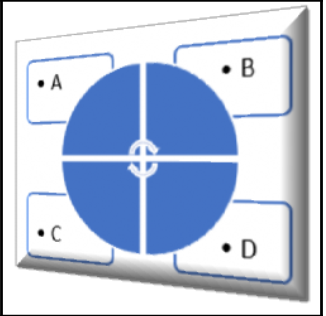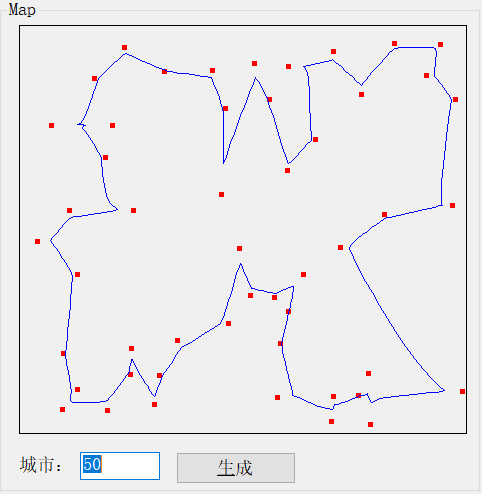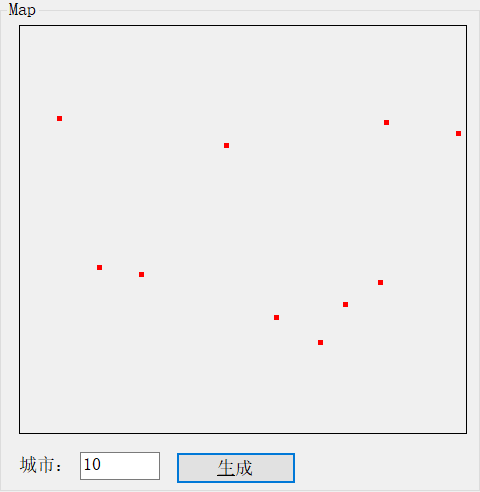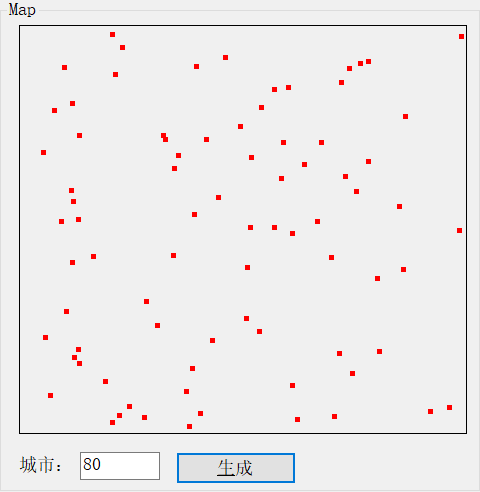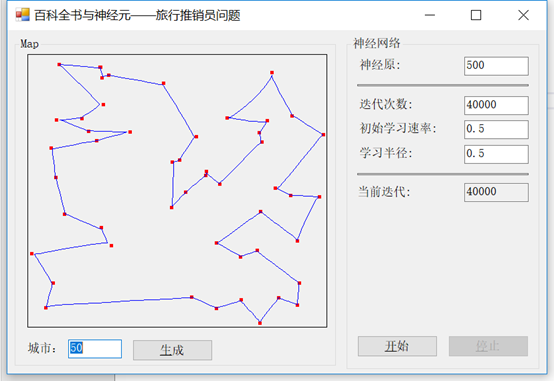/// <summary>
/// 重新生成地图
/// </summary>
private void GenerateMap()
{
Random rand = new Random((int)DateTime.Now.Ticks);

// 创建坐标数组
map = new double[citiesCount, 2];

for (int i = 0; i < citiesCount; i++)
{
map[i, 0] = rand.Next(1001);
map[i, 1] = rand.Next(1001);
}

//设置地图
chart.UpdateDataSeries("cities", map);
//删除路径
chart.UpdateDataSeries("path", null);
}

// 创建网络
DistanceNetwork network = new DistanceNetwork(2, neurons);

// 设置随机发生器范围
foreach (var neuron in network.Layers.SelectMany(layer => layer?.Neurons).Where(neuron => neuron != null))
{
neuron.RandGenerator = new UniformContinuousDistribution(new Range(0, 1000));
}

// 创建学习算法
ElasticNetworkLearning trainer = new ElasticNetworkLearning(network);

double fixedLearningRate = learningRate / 20;
double driftingLearningRate = fixedLearningRate * 19;

double[,] path = new double[neurons + 1, 2];
double[] input = new double;
int i = 0;

while (!needToStop)
{
// 更新学习速度和半径
trainer.LearningRate = driftingLearningRate * (iterations - i) / iterations + fixedLearningRate;

// 设置网络输入
int currentCity = rand.Next(citiesCount);
input = map[currentCity, 0];
input = map[currentCity, 1];

// 运行一个训练迭代
trainer.Run(input);

// 显示当前路径
for (int j = 0; j < neurons; j++)
{
path[j, 0] = network.Layers.Neurons[j].Weights;
path[j, 1] = network.Layers.Neurons[j].Weights;
}
path[neurons, 0] = network.Layers.Neurons.Weights;
path[neurons, 1] = network.Layers.Neurons.Weights;

chart.UpdateDataSeries("path", path);

i++;

SetText(currentIterationBox, i.ToString());

if (i >= iterations)
break;
}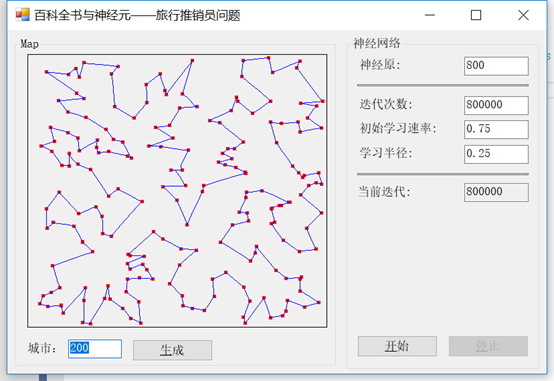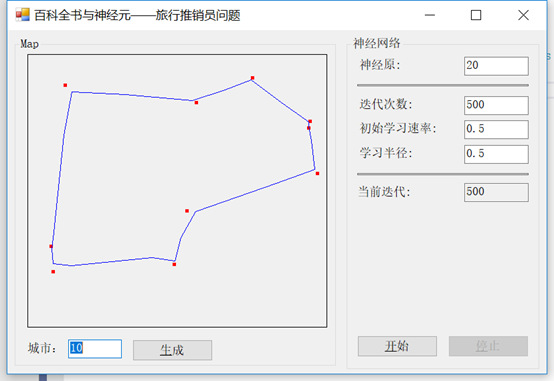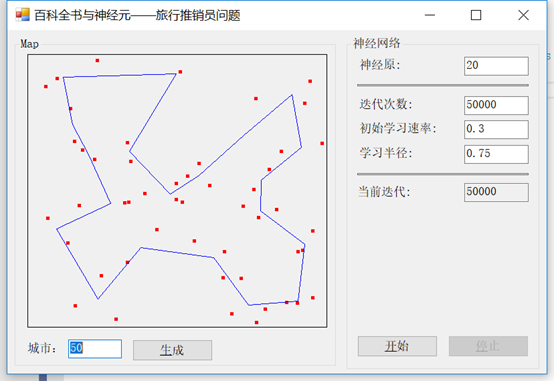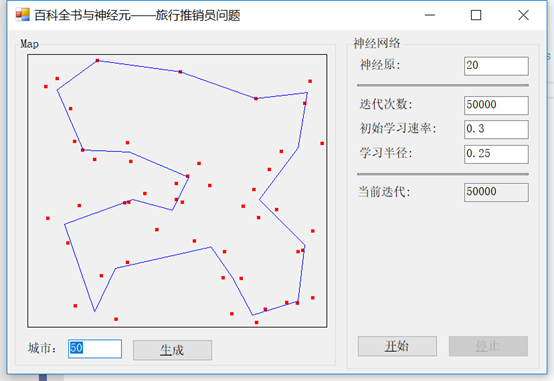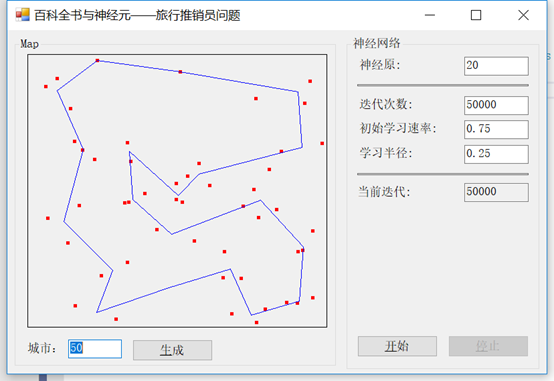// 设置随机发生器范围
foreach (var neuron in network.Layers.SelectMany(layer => layer?.Neurons).Where(neuron => neuron != null))
{
neuron.RandGenerator = new UniformContinuousDistribution(new Range(0, 1000));
}

// 创建学习算法
ElasticNetworkLearning trainer = new ElasticNetworkLearning(network);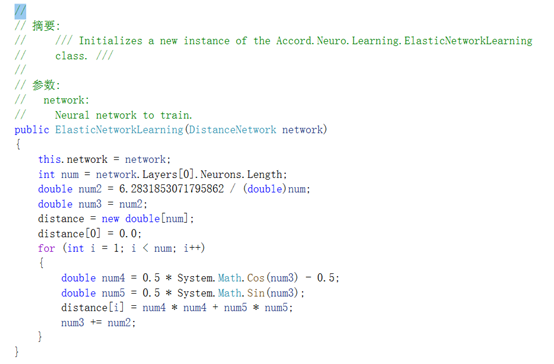double fixedLearningRate = learningRate / 20;
double driftingLearningRate = fixedLearningRate * 19;

while (!needToStop)
{
// 更新学习速度和半径
trainer.LearningRate = driftingLearningRate * (iterations - i) / iterations + fixedLearningRate;

// 设置网络输入
int currentCity = rand.Next(citiesCount);
input = map[currentCity, 0];
input = map[currentCity, 1];

// 运行一个训练迭代
trainer.Run(input);

// 显示当前路径
for (int j = 0; j < neurons; j++)
{
path[j, 0] = network.Layers.Neurons[j].Weights;
path[j, 1] = network.Layers.Neurons[j].Weights;
}
path[neurons, 0] = network.Layers.Neurons.Weights;
path[neurons, 1] = network.Layers.Neurons.Weights;

chart.UpdateDataSeries("path", path);

i++;

SetText(currentIterationBox, i.ToString());

if (i >= iterations)
break;
}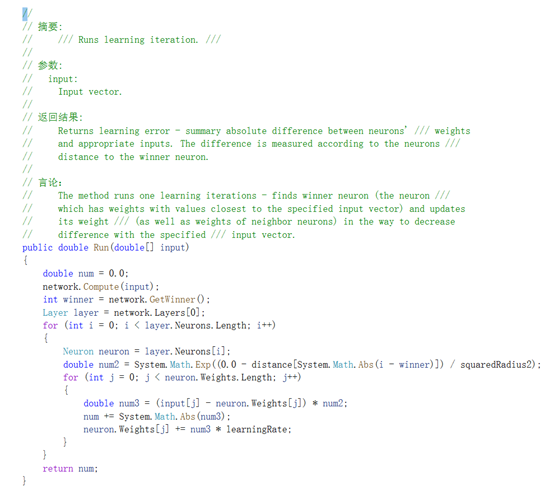## 学习速率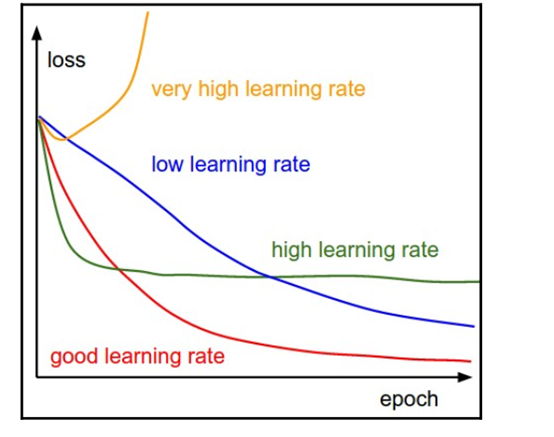## 总结

在这一章中，我们学习了神经元，还学习了著名的旅行推销员问题，它是什么，以及我们如何用电脑解决它。这个小例子在现实世界中有着广泛的应用。

在下一章中，我们将回答我们所有开发人员都面临的问题:我应该接受这份工作吗?

posted @ 2019-07-11 16:42  王振耀  阅读(...)  评论(... 编辑 收藏